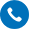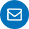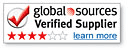Language:

### Free Samples& Design

Professional custom machined lighting accessories

•+86-13546906866
•Home / All / Industry Knowledge /

# How to calculate the lighting load?

May 29,2020
How to calculate the lighting load?
The calculation of the lighting load depends on an equation: Watt = Volt x Ampere. Mathematically written as W = VA. In most lighting situations, you will understand two of the three elements. From there, you can use the formula to find the third one. In the United States, household circuits are 120 volts. Although a few circuits may have a capacity of 240 volts, most circuits allow 15 to 20 amps. If you plan to hold a special event with lighting, or want to add special lighting to your house, you need to calculate the lighting load to ensure that it does not consume too much power and does not trip the circuit breaker.

step 1
Verify the amperage of the circuit by locating the circuit breaker panel or circuit box. The circuit breaker of each circuit should be marked with amperage, usually on the switch.

Step 2
Multiply the amperage by the voltage. For a 120V 15A circuit, the formula is as follows: W = 120 x15. The result is W = 1,800, so 1,800 watts is your maximum power load.

Step 3
Subtract the power of any other loads on the circuit (such as appliances, portable heating devices, or office equipment). The remaining wattage is the maximum load you can use for lighting.

Calculate power capacity

step 1
By adding the power of each lamp together, the load of the lamp on the circuit is calculated. For example, if you are calculating the lighting load for a special event using four 500 watt lamps, then multiply 4 by 500 to get 2,000, which is your load.

Step 2
Insert the load into the formula. In this case, you have 2,000 = VA. Since the standard household power supply is 120 volts, you can also add it to the formula to get 2,000 = 120A. In mathematical terms, 120A is the same as 120 x A. If you are on a 240 volt circuit, use 240 instead of 120.

Step 3
The amperage is calculated by dividing both sides of the equal sign by the voltage. This is done because 120 divided by 120 equals 1, and 1A is the same as A. Divide the wattage by 120 to make the equation uniform. You should end up with the following result: 16.67 = A. This means you need a circuit with 16.67 or higher amp capacity to place the load of all four lamps on that circuit. If you find that the circuit is only 15 amps, you must find a separate circuit for the fourth lamp.

*Email
Tel
*Title
*Content
• Only supports .rar/.zip/.jpg/.png/.gif/.doc/.xls/.pdf, maximum 20MB.
Contact PersonName:
Lisa Lee
Tel:
86-13528558864
86-0769-83315100
Email:
WhatsApp:
https://wa.me/8613528558864
Skype:
Skype:hongxin0713